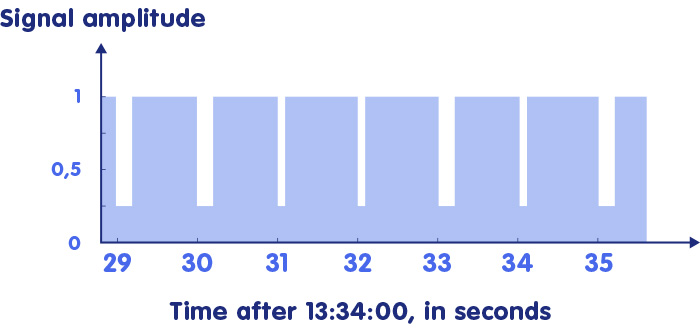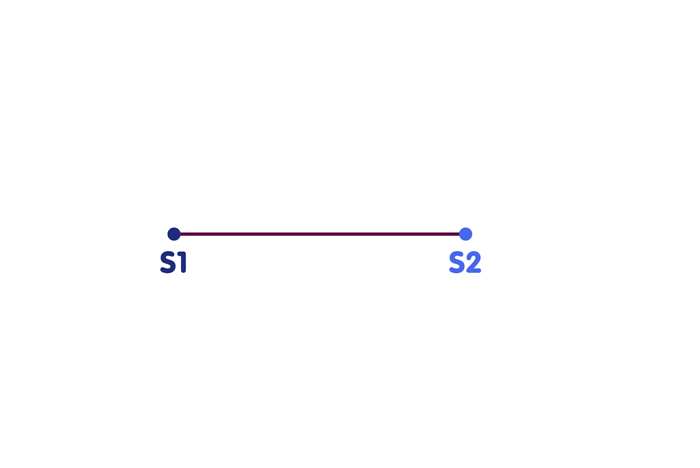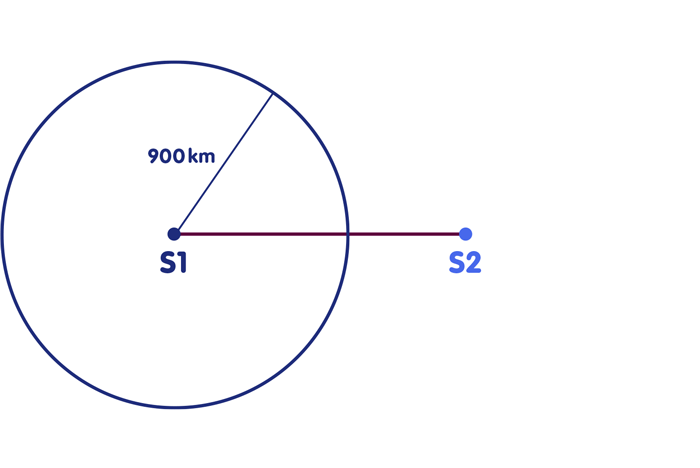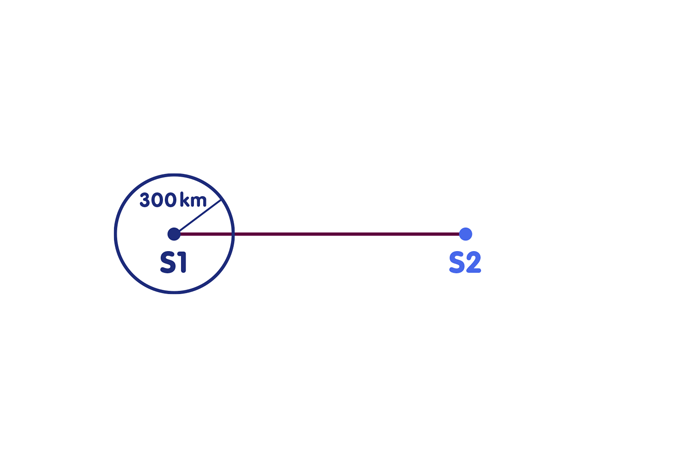### How to determine the exact time anytime and anywhere with the help of a radio receiver (and some additional information)

#### An article by Markus Pössel

Conventional radio-controlled clocks in Germany have a receiver for the time signal of the long-wave transmitter DCF77 in Mainflingen near Frankfurt am Main, which is controlled by the Physikalisch-Technische Bundesanstalt. This is a clock signal – a radio signal whose strength (more precisely, whose amplitude) periodically decreases to show that a new second has begun, as outlined here:The beginning of the drop represents the second beat. But the signal contains more information. As shown in the figure above, there are shorter and longer sinking intervals (one or two tenths of a second long). Similar to the sequence of zeros and ones in the binary code of a computer or the dot-dash sequence of a message in Morse code, this sequence of shorter and longer pulses in the course of a minute contains, among other things, the minute in which the radio station sent out the time signal in question (the radio clock must then count for itself which second in this minute it is – it recognizes the start of a new minute by the fact that the drop in signal strength stops at the fifty-ninth second). In the above example, the sequence long-long-short-short-long-short-long just indicates that the time signal refers to hour 13. The radio-controlled clock can therefore always display the correct time without having to be adjusted by hand. Switching to summer and winter time is also automatic.

## Radio-controlled clocks and the question of simultaneity

For clocks at a fixed, known location, the solution is simple. If we know where we are, and if we also know that the radio signal needs the time Δt to reach us, then it is sufficient to advance the display of our radio clock constantly by Δt, and the clock will henceforth show the correct time. In the above example: If we program our radio clock to always display the time Δt when a certain time signal t is received, where Δt is equal to one thousandth of a second, then: when the time signal 12:00:00,000 is received, our radio clock will display 12:00:00,001, which is the correct time, given the radio signal’s propagation time.

Things get a bit more difficult if we want to take our clock with us on a trip, without knowing exactly where we are at a certain time. However, this can also be remedied. For the sake of simplicity, imagine that you already know that you are on the connecting line between two radio transmitters S1 and S2, each of which transmits a time signal:Your clock is ahead, you overestimate all distances and therefore draw circles that are too large and intersect the connecting line in two different points. This is a clear indication that something went wrong, because the circles should actually intersect with the connecting line at a single point, i.e. your position.

Scenario 3 corresponds to Scenario 2, except for the difference that your clock is now not a thousandth of a second ahead, but a thousandth of a second behind. If you use your clock to infer light travel times, the rate error will cause you to underestimate all travel times by one thousandth of a second. You also underestimate all distances by the same distance, 300 kilometers, and this leads to the following wrong picture on the map:This time both circles have too small a radius. Again it becomes clear that something went wrong, because the circles intersect the connecting line at two different points instead of one.

The crucial step is that the circles not only indicate that something has gone wrong, but directly indicate by which time interval your clock is off. For one thing is characteristic for this situation: all distances are underestimated or overestimated by the same distance. If we see circles on the map, as in the figure above, whose intersections with the connecting straight line do not coincide because the radii are too small, then in our minds we can extend these radii a little by the same distance, so far that the intersections just coincide. The distance by which we had to extend both radii tells us directly by how much our clock is too slow – if we had to extend both radii by 300 kilometers, our clock one thousandth of a second behind, if 600 kilometers extension is necessary, it is two thousandths of a second behind, and so on. In exactly the same way we can shorten the radii of circles which are too large, until the points of intersection coincide, and determine from the necessary shortening, by how much our clock is ahead.

## Systematic corrections

Thus, after a necessary excursion into position determination, we are back to our initial problem, that of time determination. Because if we know by how much our clock is ahead or behind the atomic clocks at the location of the two radio clocks, we can compensate the error, and then our clock shows exactly the time of these atomic clocks.

Now we only have to draw a critical consequence from the last two scenarios: The incorrect time display on our clocks did not contribute any information to our time determination. Also for a stopped clock, we could define the correction term “indicated time minus true time” and thus determine the time of arrival of the signals. The given procedure even works if we have no clock available at our location: For two time signals from the two radio stations arriving at us at the same time, we could reconstruct when they arrive at us even without any prior clues. For let us say that the signal of the first station carries the information that it was sent out at 14:30:00,002 o’clock, that of the second station at 14:30:00,001 o’clock. We know that the two stations are 1500 kilometers apart. Suppose that the signals arrive at 14:30:00,003 o’clock. Then the first would have traveled one thousandth of a second, corresponding to 300 kilometers, the second two thousandths of a second, corresponding to 600 kilometers. In total, this adds up to 900 kilometers, much smaller than the radio station distance – so the hypothetical distance circles are much too small, our estimated time is too early. On the other hand, suppose the signals had arrived at 14:30:00.005. Then the first radio station would be 900 kilometers away, the second 1200 kilometers. The sum of the distances would clearly be larger than their true distance of 1500 kilometers – the hypothetical distance circles would be too large, the time we estimated is too late. Thus we have already narrowed down the real time. In fact, it can be determined exactly from the condition that the two distance circles intersect the connecting line in one and the same point – in our simple example even with quite simple mathematics: The calculation is exactly the same as in that notorious school problem where we have to determine at which time two trains, leaving from given stations at given times, meet on the track. If we know the arrival time of the signals, we can of course determine our location – the point where the two distance circles intersect.

In this way, satellite navigation systems such as GPS today are the most accurate way to determine time anywhere. This is related to Einstein’s theories in several ways: The basic idea is derived directly from the definition of simultaneity that Einstein introduced with his special theory of relativity. For a precise execution – as explained in the spotlight topic Relativity and Satellite Navigation – effects of both Special and General Relativity have to be taken into account. The method itself is then in turn used to research Einstein’s theory: In order to be able to compare the data of the various gravitational wave detectors worldwide, their temporal evolution is precisely logged – the highly precise time required for this is provided by the satellite navigation system GPS.

## Further Information

The basic relativistic concepts underlying this spotlight topic are explained in Elementary Einstein, in particular in the section on special relativity.

Related spotlight topics on Einstein-Online can be found in the categories Special Relativity and General Relativity.

More about time determination with radio clocks can be found on the web pages of the working group “Dissemination of Time” of the Physikalisch-Technische Bundesanstalt.

###### Colophon
Markus Pössel

is the managing scientist at Haus der Astronomie, the Center for Astronomy Education and Outreach in Heidelberg, and senior outreach scientist at the Max Planck Institute for Astronomy. He initiated Einstein Online.